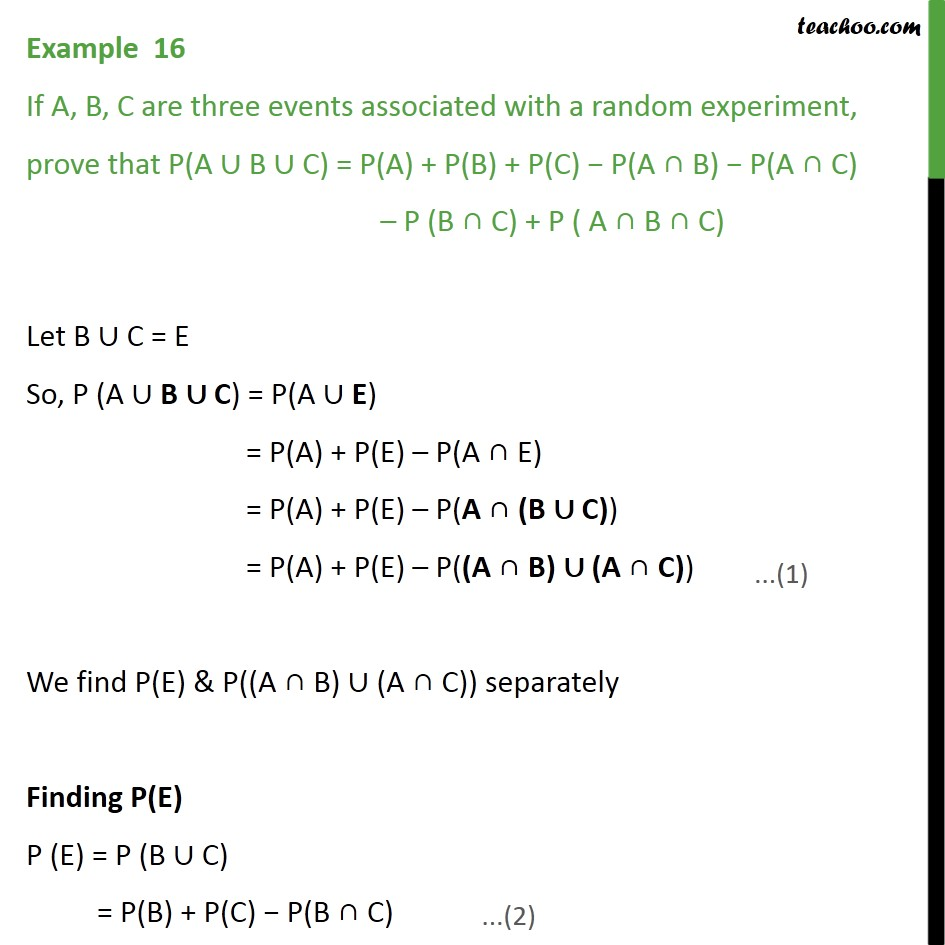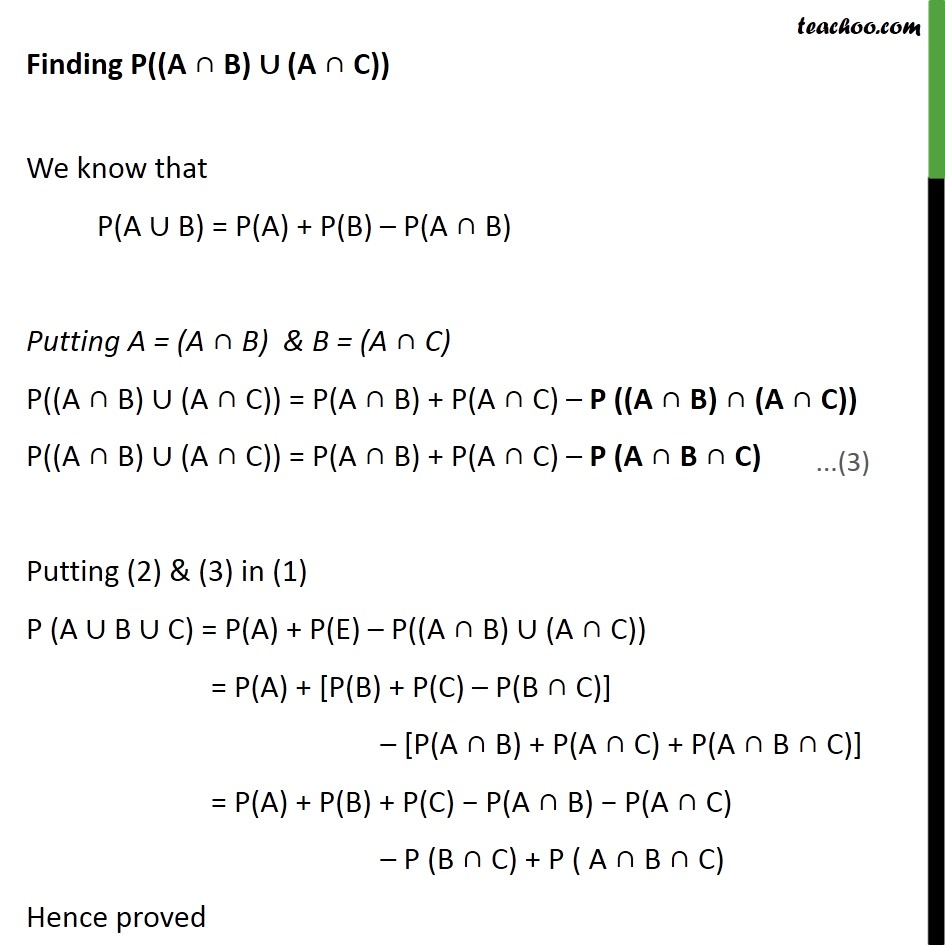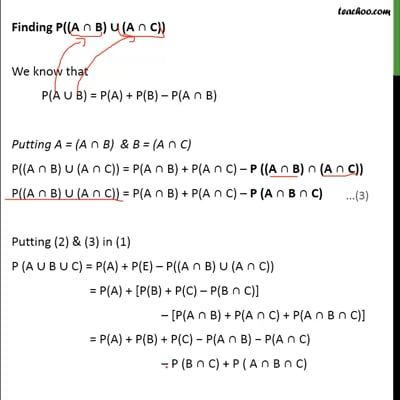Examples

Chapter 14 Class 11 Probability
Serial order wiseThis video is only available for Teachoo black users

Learn in your speed, with individual attention - Teachoo Maths 1-on-1 Class

### Transcript

Example 11 If A, B, C are three events associated with a random experiment, prove that P(A ∪ B ∪ C) = P(A) + P(B) + P(C) − P(A ∩ B) − P(A ∩ C) – P (B ∩ C) + P ( A ∩ B ∩ C) Let B ∪ C = E So, P (A ∪ B ∪ C) = P(A ∪ E) = P(A) + P(E) – P(A ∩ E) = P(A) + P(E) – P(A ∩ (B ∪ C)) = P(A) + P(E) – P((A ∩ B) ∪ (A ∩ C)) We find P(E) & P((A ∩ B) ∪ (A ∩ C)) separately Finding P(E) P (E) = P (B ∪ C) = P(B) + P(C) − P(B ∩ C) Finding P((A ∩ B) ∪ (A ∩ C)) We know that P(A ∪ B) = P(A) + P(B) – P(A ∩ B) Putting A = (A ∩ B) & B = (A ∩ C) P((A ∩ B) ∪ (A ∩ C)) = P(A ∩ B) + P(A ∩ C) – P ((A ∩ B) ∩ (A ∩ C)) P((A ∩ B) ∪ (A ∩ C)) = P(A ∩ B) + P(A ∩ C) – P (A ∩ B ∩ C) Putting (2) & (3) in (1) P (A ∪ B ∪ C) = P(A) + P(E) – P((A ∩ B) ∪ (A ∩ C)) = P(A) + [P(B) + P(C) – P(B ∩ C)] – [P(A ∩ B) + P(A ∩ C) + P(A ∩ B ∩ C)] = P(A) + P(B) + P(C) − P(A ∩ B) − P(A ∩ C) – P (B ∩ C) + P ( A ∩ B ∩ C) Hence proved